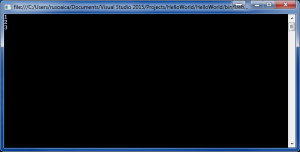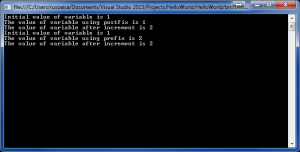Wednesday, December 06, 2023 04:46

## Incrementing operator

One of the most used operations in programming is incrementing a variable’s value. Incrementing operator is composed of two plus signs: ++ (and has two forms), or a variation of the following two operators: += and =+.

Lets consider the following example:

In the above example, we are assigning a variable the result of adding 1 to its own value. That is called increment by 1Because incrementing operation is so common in software, programming languages, including C#, offer the programmers a short notation for it, named incrementing operator. The next piece of code uses both operators of incrementing a variable:

The output will be the following:As you can see, we are declaring an initializing an integer variable, with a value of 1. After that, the variable is incrementing in both examples by 1.

The difference between variable++ and variable += 1 is that in the first case, the variable is always incremented by 1. In the second case, we can increment it by whatever value we want, like in the following example:

As you will see, the incrementing operator will often be used in pieces of code where the program is repeating the same instructions a number of times, called loops (we will learn about them later on).

If you noticed at the beginning of this lesson, I said that the ++ variation of the incrementing operator has two forms, and I also said that you can have += or =+. What is the difference between these two concepts? They are distinguished as prefix and postfixLets take the following piece of code:

The difference between prefix and postfix is that when you are using the postfix incrementing operator, C# will use the value of the variable first, and only after that will increment its value. On the other side, when using the prefix incrementing operator, C# will increment the value first, and then use its value.  The following example will illustrate the difference:

And the output will beNow you can notice that when you use the postfix incrementing operator, C# uses the value of the variable first, displaying 1, then increments it, displaying 2. On the other hand, using the prefix incrementing operator, C# increments the variable first, displaying 2, then it uses and displays the value already incremented.

The concepts explained in this lesson are also shown visually as part of the following video: